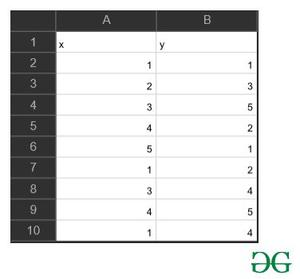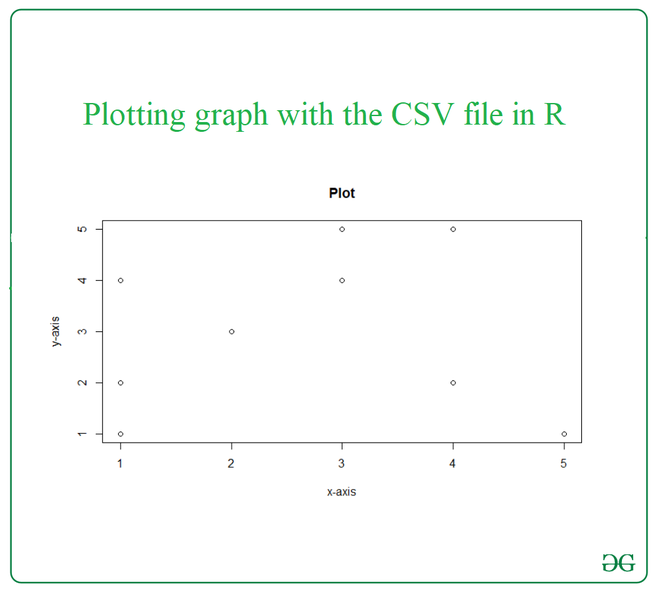# How to plot a graph in R using CSV file ?

• Difficulty Level : Basic
• Last Updated : 26 Mar, 2021

To plot a graph in R using a CSV file, we need a CSV file with two-column, the values in the first column will be considered as the points at the x-axis and the values in the second column will be considered as the points at the y-axis. In this article, we will be looking at the way to plot a graph using a CSV file in R language.

Approach

• Import csv file
• Pass required parameters to plot function
• Plot graph
• Display plot

Functions Used:

• To import/read the CSV file to the R console, the user must need to use the read.csv() function in R.This function will read a CSV file available in your current working directory.

Syntax:

• plot() function for plotting of R objects. With the provided parameters this function returns a scatter plot by default.

Syntax:

plot(x,y,main,xlab,ylab,sub,asp)

Parameters:

• x:-the x coordinates of points in the plot
• y:-the y coordinates of points in the plot
• main:-an overall title for the plot
• sub:-a subtitle for the plot
• xlab:-a title for the x-axis
• ylab:-a title for the y-axis
• asp:-the y/x aspect ratio

Return:

Scatter plot of the given x and y values.Example:

## R

 `data=``read.csv``(``'input_gfg.csv'``)`` ` `print``(data)`` ` `plot``(x = data\$x,y = data\$y,``     ``xlab = ``"x-axis"``,``     ``ylab = ``"y-axis"``,``     ``main = ``"Plot"``)`

Output:My Personal Notes arrow_drop_up